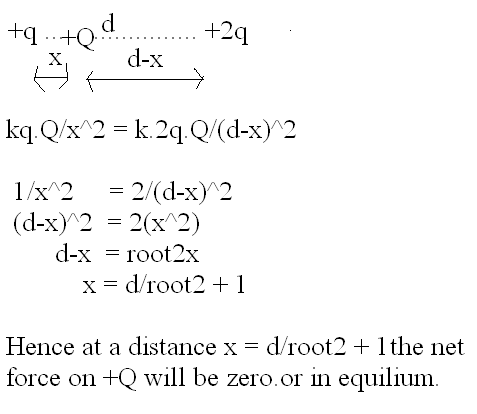# Q. Two point electric charges of values q and 2q are kept at a distance d apart from each other in air. A third charge Q is to be kept along the same line in such a way that the net force acting on q and 2q is zero. Calculate the position of charge Q in terms of q and d...??? plz explain me in brief...

9 years ago

the given situation is:

F2q <———q———>FQ----------Q------------------------FQ<———2q———>Fq

d-x                    x

now if net force on 2q has to be 0 then Q has to be negative and hence 2q will experience a force towards Q(attraction).But 2q will also experience a force away from q(repulsion).Hence these forces can be calculated using coulombs law and equated and get x.Same thing can be done with q to get another equation and using the two equation you can eliminate Q.

Hence,

FQ=Fq          and             F2q=FQ

9 years agoPLZ  APPROVE!

9 years ago

q and 2q are d distance apart.

let charge Q be kept between them on the same line joining those two ..at a distance of x from q.

now a repulsive force will be acting on it by q as well as by 2q but in opp. directions.

( if q at left and 2q at right), then

forcE on Q by q=  k (qQ/x^2)         towards right

"     "   Q by 2q = k((2qQ/(d-x)^2)    "    left

since these 2 forces should be equal and opp. for net force on Q to be zero,

so   by equating tjose two,

we get

x=[d(q+q^1/2)]/(q-2)        or  [d(q-q^1/2)]/(q-2)Kushagra Madhukar
2 years ago
Dear student,
F2q ← q → FQ                      Q                           FQ2q → Fq
x                      d – x
Now, Net force on q = F2q + FQ = 0
Hence, kq(2q)/d2 + kqQ/x2 = 0
or, Q = – 2qx2/d2

Net force on 2q is also zero.
Hence, Fq + FQ = 0
Hence, kq(2q)/d2 + k(2q)Q/(d – x)2 = 0
Hence, q/d2 + ( – 2qx2/d2)/(d – x)2 = 0
or, (d – x)2 – 2x2 = 0
or, d2 + x2 – 2xd – 2x2 = 0
or, x2 + 2xd – d2 = 0
The positive root of this equation is,
x = d / (√2 + 1)

Hope it helps.
Thanks and regards,
Kushagra